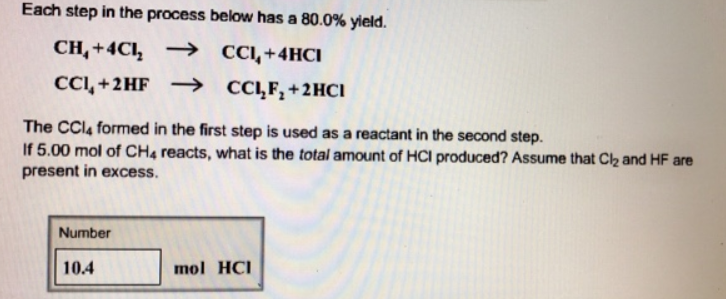# Problem: Each step in the process below has a 80.0% yield. The CCI4 formed in the first step is used as a reactant in the second step. If 5.00 mol of CH4 reacts, what is the total amount of HCI produced? Assume that Cl2 and HF are present in excess.

###### FREE Expert Solution
88% (113 ratings)###### Problem Details

Each step in the process below has a 80.0% yield.

The CCI4 formed in the first step is used as a reactant in the second step. If 5.00 mol of CH4 reacts, what is the total amount of HCI produced? Assume that Cl2 and HF are present in excess.Frequently Asked Questions

What scientific concept do you need to know in order to solve this problem?

Our tutors have indicated that to solve this problem you will need to apply the Percent Yield concept. You can view video lessons to learn Percent Yield. Or if you need more Percent Yield practice, you can also practice Percent Yield practice problems.

What professor is this problem relevant for?

Based on our data, we think this problem is relevant for Professor Tesema's class at NVCC.